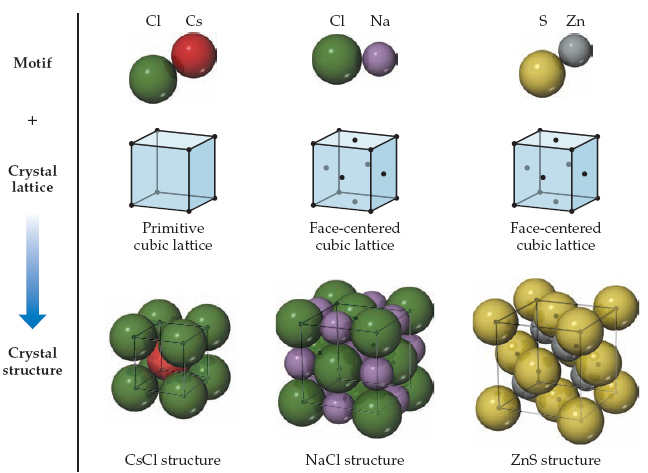# Problem:  The structures of CsCl, NaCl, and ZnS. Each structure type can be generated by the combination of a two-atom motif and the appropriate lattice.CuI, CsI, and NaI each adopt a different type of structure. The three different structures are those shown in the following figure.Write the coordination numbers of iodide in CuI, CsI, and NaI, respectively.

###### FREE Expert Solution

We're being asked to determine the coordination number of iodide in the given compounds.

The coordination number is the number of ions that immediately surround an ion of the opposite charge within a crystal lattice.

Crystalline solids represent structures with well-organized patterns and shapes. When examining the particles within a crystal you may observe them tightly packed in an organized pattern. The smallest portion of which is termed the unit cell.

• The Simple Cubic Unit Cell is composed of cube with an atom at each corner. An atom in a simple cubic lattice structure contacts six other atoms, so its coordination number is six (6).
• The body-centered cubic unit cell is composed of a cube with one atom at each of its corners and one atom at the center of the cube. Any atom in the structure touches four atoms in the layer above it and four atoms in the layer below it so its coordination number is eight (8).
• The face-centered cubic unit cell is composed of a cube with one atom at each of its corners and one atom in the center of each cube face. Atoms in this structure contact six atoms in their layer, three atoms in the layer above it and three atoms in the layer below it so its coordination number is twelve (12).

Let’s analyze the problem using the information we have###### Problem DetailsThe structures of CsCl, NaCl, and ZnS. Each structure type can be generated by the combination of a two-atom motif and the appropriate lattice.

CuI, CsI, and NaI each adopt a different type of structure. The three different structures are those shown in the following figure.

Write the coordination numbers of iodide in CuI, CsI, and NaI, respectively.